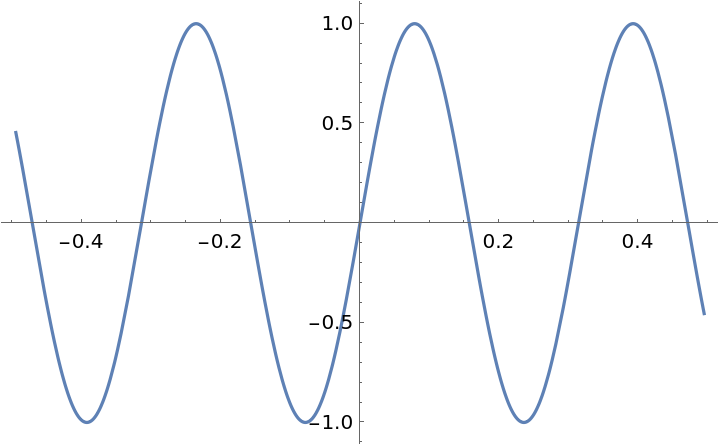#Function Repository Resource:

# SuggestPlotRange

Get a variable range against which to plot a given function

Contributed by: Wolfram|Alpha Math Team
 ResourceFunction["SuggestPlotRange"][expr,x] tries to return a range in x that will produce a nice-looking plot of the expression expr versus x.

## Details and Options

ResourceFunction["SuggestPlotRange"][expr, x] returns a result of the form {x, xmin, xmax} that can be used directly as a range specifier in Plot.
ResourceFunction["SuggestPlotRange"] takes the following options:
 "Periods" Automatic how many periods of a periodic function to plot "Range" "Narrow" whether to give a "Narrow" or "Wide" plot range

## Examples

### Basic Examples (5)

Suggest a plot range for a trigonometric function:

 In:=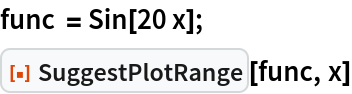Out=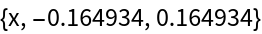In:=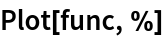Out=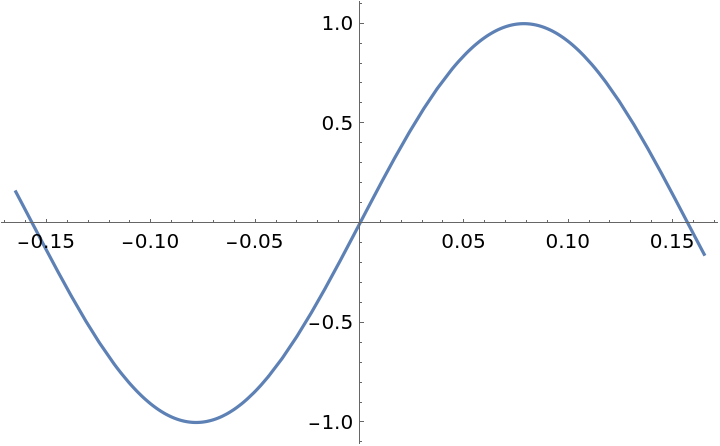Suggest a plot range for a polynomial function:

 In:=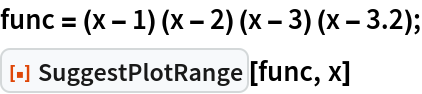Out=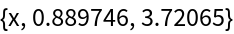In:=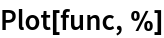Out=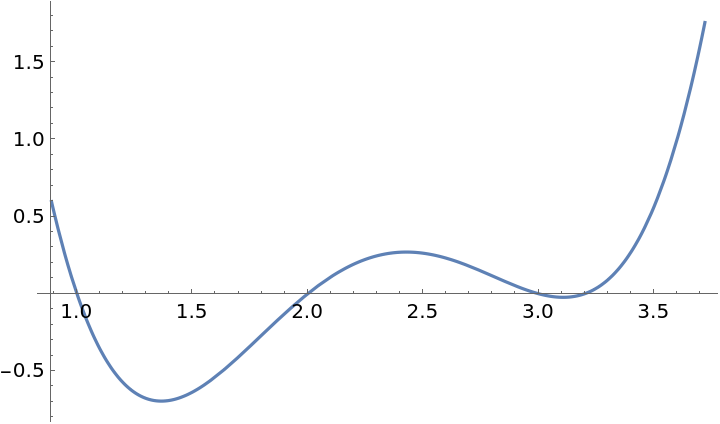Suggest a plot range for a rational function:

 In:=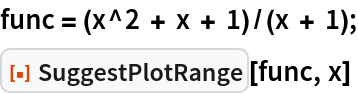Out=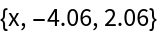In:=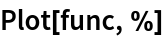Out=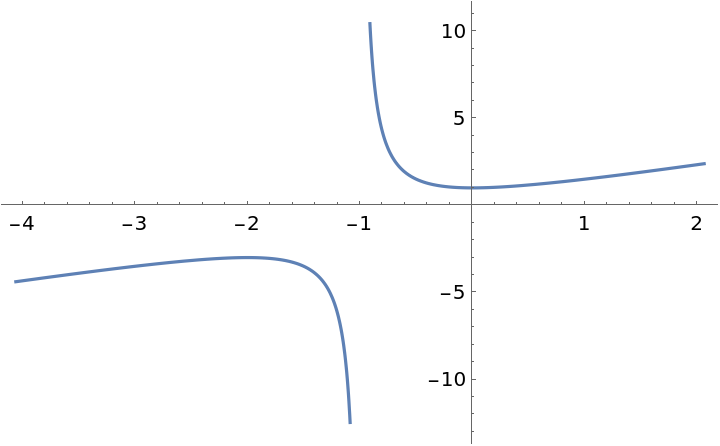Suggest a plot range for a generic function:

 In:=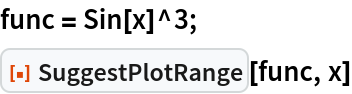Out=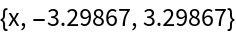In:=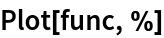Out=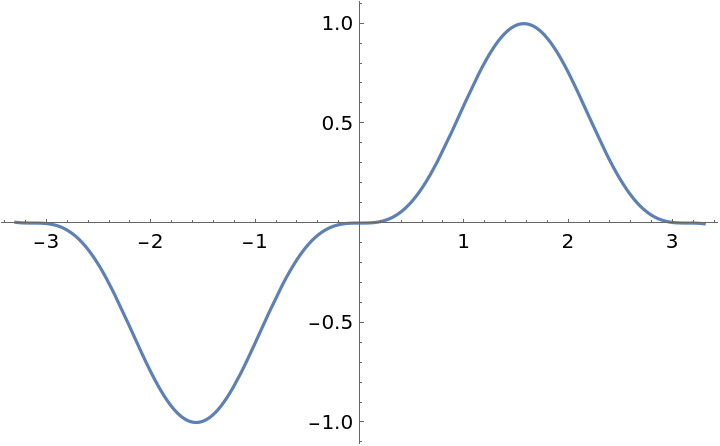Suggest a plot range for another function:

 In:=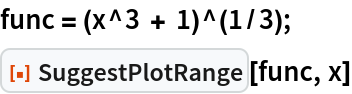Out=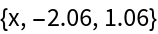In:=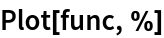Out=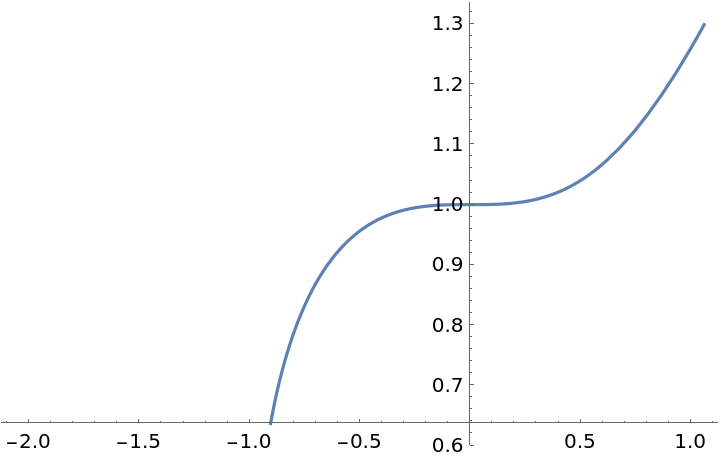### Options (2)

Using the option setting Range -> "Wide" will give a range showing more of the function but possibly revealing less detail:

 In:=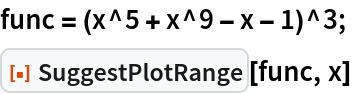Out=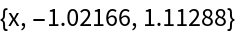In:=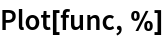Out=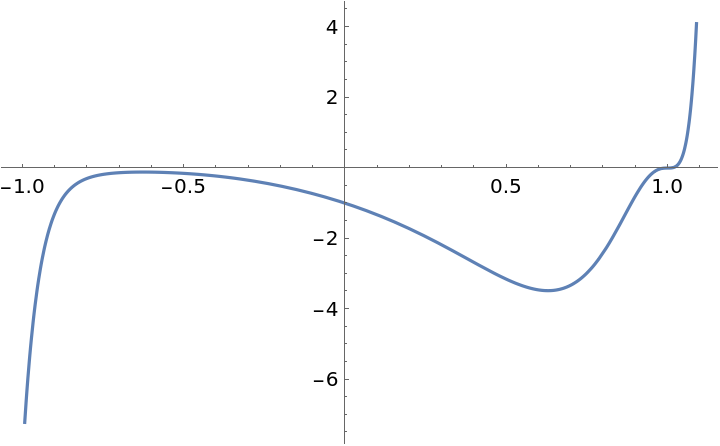In:=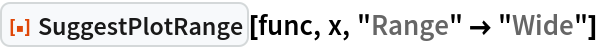Out=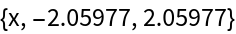In:=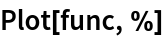Out=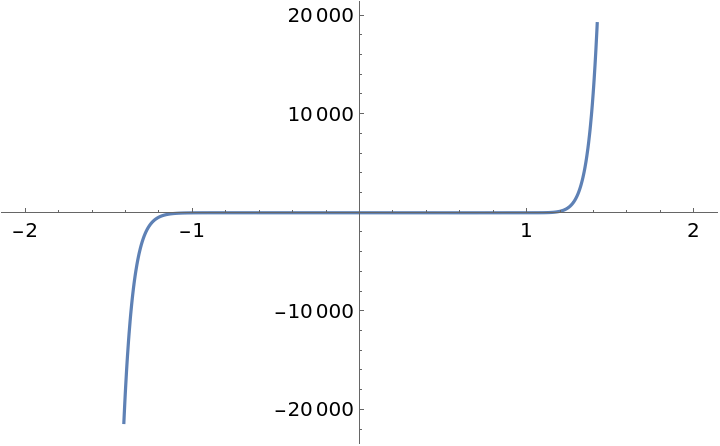For periodic functions, the option setting "Periods"n will return a range covering the specified number of periods:

 In:=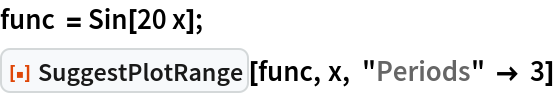Out=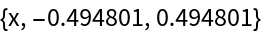In:=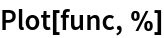Out=